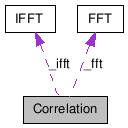# Correlation Class Reference

Algorithm to perform the correlation between two vectors of Real values. More...

`#include <Correlation.h>`

Collaboration diagram for Correlation:[legend]

List of all members.

## Public Member Functions

Correlation (int inputSizeA, int inputSizeB, int maxLag, int minLag, bool useFft)
~Correlation ()
void process (const MatrixXR &framesA, const MatrixXR &framesB, MatrixXR *correlation)
int inputSizeA () const
void setInputSizeA (int size, bool callSetup=true)
int inputSizeB () const
void setInputSizeB (int size, bool callSetup=true)
int minLag () const
void setMinLag (int lag, bool callSetup=true)
int maxLag () const
void setMaxLag (int lag, bool callSetup=true)
bool useFft () const
void setUseFft (bool useFft, bool callSetup=true)

## Detailed Description

Algorithm to perform the correlation between two vectors of Real values.

This class represents an object to perform a correlation of two vectors.

The correlation can be performed using two methods:

1. Direct method
2. FFT method

The Direct method consists in applying the correlation formula directly in the time domain.

The FFT method consists in performing an Fast Fourier Transform of each of the vectors and multiply the first by the conjugate of the second. Finally the algorithm applies an IFFT to the result of the multiplication in order to obtain the autocorrelation for all the time lags.

The Direct method performs faster than the FFT method only on vectors of small sizes. The decision point for selecting one of the two methods depends on the platform.

The method performed can be specified using setUseFft().

Autocorrelation

## Constructor & Destructor Documentation

 Correlation ( int inputSizeA, int inputSizeB, int maxLag, int minLag, bool useFft )

Constructs an Autocorrelation object with the specified inputSize, maxLag and minLag settings.

Parameters:
 inputSizeA size of the first input arrays to be autocorrelated, must be > 0. The algorithm performs faster for sizes which are a power of 2. inputSizeB size of the second input arrays to be autocorrelated, must be > 0. The algorithm performs faster for sizes which are a power of 2. maxLag maximum lag to be calculated minLag minimum lag to be calculated useFft determines whether or not to use the FFT method

References setInputSizeA(), setInputSizeB(), setMaxLag(), setMinLag(), and setUseFft().

 ~Correlation ( )

Destroys the Correlation algorithm and frees its resources.

## Member Function Documentation

 void process ( const MatrixXR & framesA, const MatrixXR & framesB, MatrixXR * correlation )

Performs a Correlation between each of the rows of framesA and each of the rows of framesB respectively. Puts the resulting correlations in the rows of correlation.

Parameters:
 framesA matrix of Real values. The number of columns of framesA must be equal to the inputSizeA property. framesB matrix of Real values. The number of columns of framesB must be equal to the inputSizeB property.
Note that framesA and framesB should have the same number of rows.

Parameters:
 correlation pointer to a matrix of Real values for the output. The matrix should have the same number of rows as framesA (and framesB) and maxLag - minLag columns.
Note that if the output matrix is not of the required size it will be resized, reallocating a new memory space if necessary.

References IFFT::process(), and FFT::process().

 int inputSizeA ( ) const

Returns the size of the first input arrays to be correlated. The default is 1024.

inputSizeB(), setInputSizeA(), setInputSizeB()

 void setInputSizeA ( int size, bool callSetup = `true` )

Specifies the size of the first of the input arrays. The given size must be higher than 0. Note that if size is a power of 2 the algorithm will perform faster.

inputSizeA(), inputSizeB(), setInputSizeB()

Referenced by Correlation().

 int inputSizeB ( ) const

Returns the size of the second of the input arrays to be correlated. The default is 1024.

inputSizeA(), setInputSizeA(), setInputSizeB()

 void setInputSizeB ( int size, bool callSetup = `true` )

Specifies the size of the second of the input arrays. The given size must be higher than 0. Note that if size is a power of 2 the algorithm will perform faster.

inputSizeA(), inputSizeB(), setInputSizeA()

Referenced by Correlation().

 int minLag ( ) const

Returns the minimum lag to be calculated. The default is -max(_inputSizeA, _inputSizeB) + 1.

maxLag(), setMinLag(), setMaxLag()

 void setMinLag ( int lag, bool callSetup = `true` )

Specifies the minimum lag of the ocorrelation. The given lag will be constratined between - max( inputSizeA, inputSizeB ) + 1 and min( inputSizeA, inputSizeB ). Note that the lag should be smaller than the maxLag.

minLag(), maxLag(), setMaxLag()

Referenced by Correlation().

 int maxLag ( ) const

Returns the maximum lag to be calculated. The default is inputSize.

minLag(), setMinLag(), setMaxLag()

 void setMaxLag ( int lag, bool callSetup = `true` )

Specifies the maximum lag of the correlation. The given lag will be constratined between - max( inputSizeA, inputSizeB ) + 1 and min( inputSizeA, inputSizeB ).

Note that the lag should be larger than the maxLag.

minLag(), maxLag(), setMinLag()

Referenced by Correlation().

 bool useFft ( ) const

Returns `true` if the FFT method should be used for the correlation. The default is True for inputSize larger than 128; otherwise it is False.

setUseFft()

 void setUseFft ( bool useFft, bool callSetup = `true` )

Specifies whether the autocorrelation should be performed using the FFT method.

Generated on Tue Mar 31 20:38:33 2009 for Loudia by1.5.6